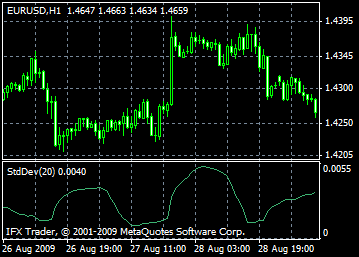# Standard Deviation indicator: description, adjustment and application

Standard Deviation (StdDev) is a technical indicator that is used for determination of trend and market volatility. This indicator measures range of fluctuations against the moving average. Standard Deviation is often used as a part of other technical indicators.

For example, when calculating Bollinger Bands, you add value of Standard Deviation to the moving average.

The market can be considered volatile if the value of the indicator is high and the prices of bars vary and are scattered far from the moving average. If the market is flat, then the prices of bars are close to the moving average, which indicate low volatility.

Price movements sequentially switch from periods of ease to bursts of activity and back, so the strategy for analysis of the Standard Deviation indicator is simple. If the value of the indicator is too low, that is, the market is flat, then you should expect a spike in activity. And vice versa, if the indicator shows an extremely high value, then quite soon the market will descend to a state of rest.#### Calculation

StdDev (i) = SQRT (AMOUNT (j = i - N, i) / N)

AMOUNT (j = i - N, i) = SUM ((ApPRICE (j) - MA (ApPRICE (i), N, i)) ^ 2), where:

StdDev (i) — standard deviation of the current bar;

SQRT — square root;

AMOUNT(j = i - N, i) — sum of squares of j = i - N to i;

N — period of smoothing;

ApPRICE (j) — applied price of the j bar;

MA (ApPRICE (i), N, i) — any moving average of the current bar for the N periods;

ApPRICE (i) — applied price of the current bar.

Can't speak right now?